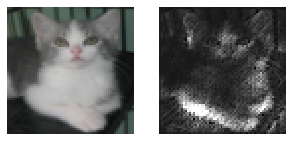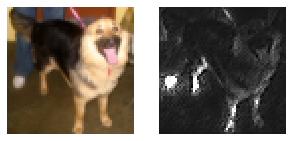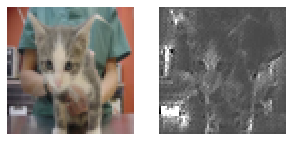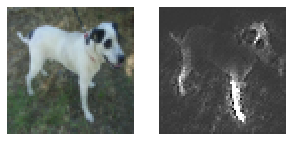Deep Learning Models -- A collection of various deep learning architectures, models, and tips for TensorFlow and PyTorch in Jupyter Notebooks.

In :
%load_ext watermark
%watermark -a 'Sebastian Raschka' -v -p torch

Sebastian Raschka

CPython 3.7.3
IPython 7.9.0

torch 1.4.0

• Runs on CPU or GPU (if available)

# Guided Backpropagation¶

## (Based on a VGG16 Convolutional Neural Network for Kaggle's Cats and Dogs Images)¶

Vizualization of the loss gradients with respect to the inputs (images) as a naive way to visualize CNN predictions. I.e.,

$$\nabla L(\mathbf{x})= \left[\begin{array}{c} \frac{\partial L}{\partial x_1} \\ \frac{\partial L}{\partial x_2} \\ \vdots \end{array}\right]$$

via guided backpropagation . In a normal forward pass, negative activation values are clamped by ReLU functions. Consequently, the gradient of these clamped values are 0 as well. In guided backpropagation, we go a step further and also clamp the negative gradients during backpropagation to 0. The rationale behind this is that setting negative gradients to 0 will focus only on those activations that have a positive influence on the class of interest.

• Springenberg, J. T., Dosovitskiy, A., Brox, T., & Riedmiller, M. (2014). Striving for simplicity: The all convolutional net. arXiv preprint arXiv:1412.6806.

Please note that this notebook is to be run after ./_cnn-basemodel__vgg16-cats-dogs.ipynb, which trains the VGG16 model on thh cats and dogs dataset.

## Imports¶

In :
import time
import os

import numpy as np

import torch
import torch.nn.functional as F
import torch.nn as nn

from torchvision import transforms

from PIL import Image
import matplotlib.pyplot as plt

if torch.cuda.is_available():
torch.backends.cudnn.deterministic = True

In :
%matplotlib inline


## Settings¶

In :
##########################
### SETTINGS
##########################

# Device
DEVICE = torch.device("cuda:0" if torch.cuda.is_available() else "cpu")

# Hyperparameters
RANDOM_SEED = 1
BATCH_SIZE = 128

# Architecture
NUM_CLASSES = 2

# Image normalization parameters from _cnn-basemodel__vgg16-cats-dogs.ipynb
train_mean = [0.4875, 0.4544, 0.4164]
train_std = [0.2521, 0.2453, 0.2481]


## Cats vs Dogs Dataset¶

In :
img = Image.open(os.path.join('dogs-vs-cats','train', 'cat.59.jpg'))
print(np.asarray(img, dtype=np.uint8).shape)
plt.imshow(img);

(331, 464, 3)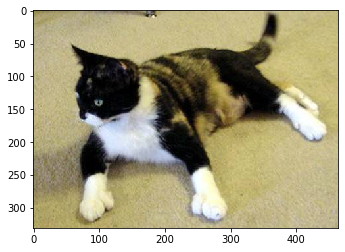In :
from datautils import CatsDogsDataset

data_transforms = {
'train': transforms.Compose([
transforms.RandomRotation(5),
transforms.RandomHorizontalFlip(),
transforms.RandomResizedCrop(64, scale=(0.96, 1.0), ratio=(0.95, 1.05)),
transforms.ToTensor(),
transforms.Normalize(train_mean, train_std)
]),
'valid': transforms.Compose([
transforms.Resize([64, 64]),
transforms.ToTensor(),
transforms.Normalize(train_mean, train_std)
]),
}

batch_size=BATCH_SIZE,
data_transforms=data_transforms,
train_path=os.path.join('dogs-vs-cats', 'train'),
valid_path=os.path.join('dogs-vs-cats', 'valid'),
test_path=os.path.join('dogs-vs-cats', 'test')
)


## Model¶

In :
from vgg16 import VGG16

In :
torch.manual_seed(RANDOM_SEED)
model = VGG16(num_classes=NUM_CLASSES)
model = model.to(DEVICE)


Out:
<All keys matched successfully>

### Preprocessing Code¶

In :
from datautils import UnNormalize

unorm = UnNormalize(mean=train_mean, std=train_std)

def preprocess_image(image_path, transform_func, unnormalize_func):

pil_img = Image.open(image_path)
img_tensor = transform_func(pil_img)
unnormed_img_tensor = unnormalize_func(img_tensor)

return pil_img, img_tensor, unnormed_img_tensor

In :
def get_viz(image_tensor, label, model, device=DEVICE):

image_tensor = image_tensor.to(device)
label = torch.tensor(label).to(device)
# convert CHW tensor to NCHW tensor
# note that N=1
image_tensor = image_tensor.unsqueeze(0)

### FORWARD AND BACK PROP
logits, probas = model(image_tensor)
cost = F.cross_entropy(logits, label)
cost.backward()

# get CHW tensor from NCHW tensor
# note that N=1

In :
# Simple Hook class that records the input and output of a layer during forward/backward pass

class GuidedBackprop():
def __init__(self, model, device):
self.model = model
self.device = device

def setup_hooks(self):

for module in self.model.modules():
if type(module) == nn.ReLU:
module.register_backward_hook(relu_backward_hook_fn)

def run(self, image_tensor, label):
image_tensor = image_tensor.to(self.device)
label = torch.tensor([label]).to(self.device)
# convert CHW tensor to NCHW tensor
# note that N=1
image_tensor = image_tensor.unsqueeze(0)

### FORWARD AND BACK PROP
logits, probas = self.model(image_tensor)
cost = F.cross_entropy(logits, label)
cost.backward() # computes gradients via hook


In :
guidedbackprop = GuidedBackprop(model, DEVICE)
guidedbackprop.setup_hooks()

In :
from datautils import convert_rgb_to_grayscale

model.eval()

for img_path, lab in zip(['cat.11252.jpg', 'dog.12018.jpg', 'cat.11267.jpg', 'dog.12077.jpg'],
[0, 1, 0, 1]):

pil_img, img_tensor, unnormed_img_tensor = preprocess_image(
os.path.join('./dogs-vs-cats/test/', img_path),
data_transforms['valid'],
unorm
)

# visualize results
fig, ax = plt.subplots(1, 2, figsize=(5, 10))

# plot original
ax.imshow(np.transpose(unnormed_img_tensor.cpu().numpy(), (1, 2, 0)))
ax.axis('off')